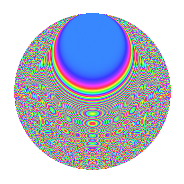# Properties

 Label 8006.2.aLevel 8006 Weight 2 Character orbit a Rep. character $$\chi_{8006}(1,\cdot)$$ Character field $$\Q$$ Dimension 334 Newforms 4 Sturm bound 2002 Trace bound 1

# Related objects

## Defining parameters

 Level: $$N$$ = $$8006 = 2 \cdot 4003$$ Weight: $$k$$ = $$2$$ Character orbit: $$[\chi]$$ = 8006.a (trivial) Character field: $$\Q$$ Newforms: $$4$$ Sturm bound: $$2002$$ Trace bound: $$1$$

## Dimensions

The following table gives the dimensions of various subspaces of $$M_{2}(\Gamma_0(8006))$$.

Total New Old
Modular forms 1003 334 669
Cusp forms 1000 334 666
Eisenstein series 3 0 3

The following table gives the dimensions of the cuspidal new subspaces with specified eigenvalues for the Atkin-Lehner operators and the Fricke involution.

$$2$$$$4003$$FrickeDim.
$$+$$$$+$$$$+$$$$75$$
$$+$$$$-$$$$-$$$$92$$
$$-$$$$+$$$$-$$$$98$$
$$-$$$$-$$$$+$$$$69$$
Plus space$$+$$$$144$$
Minus space$$-$$$$190$$

## Trace form

 $$334q$$ $$\mathstrut +\mathstrut 334q^{4}$$ $$\mathstrut -\mathstrut 4q^{5}$$ $$\mathstrut +\mathstrut 2q^{6}$$ $$\mathstrut +\mathstrut 340q^{9}$$ $$\mathstrut +\mathstrut O(q^{10})$$ $$334q$$ $$\mathstrut +\mathstrut 334q^{4}$$ $$\mathstrut -\mathstrut 4q^{5}$$ $$\mathstrut +\mathstrut 2q^{6}$$ $$\mathstrut +\mathstrut 340q^{9}$$ $$\mathstrut -\mathstrut 6q^{10}$$ $$\mathstrut +\mathstrut 2q^{11}$$ $$\mathstrut +\mathstrut 6q^{13}$$ $$\mathstrut +\mathstrut 334q^{16}$$ $$\mathstrut -\mathstrut 12q^{17}$$ $$\mathstrut +\mathstrut 10q^{19}$$ $$\mathstrut -\mathstrut 4q^{20}$$ $$\mathstrut +\mathstrut 4q^{22}$$ $$\mathstrut -\mathstrut 8q^{23}$$ $$\mathstrut +\mathstrut 2q^{24}$$ $$\mathstrut +\mathstrut 328q^{25}$$ $$\mathstrut -\mathstrut 4q^{26}$$ $$\mathstrut -\mathstrut 6q^{29}$$ $$\mathstrut +\mathstrut 12q^{30}$$ $$\mathstrut -\mathstrut 20q^{33}$$ $$\mathstrut +\mathstrut 8q^{34}$$ $$\mathstrut -\mathstrut 24q^{35}$$ $$\mathstrut +\mathstrut 340q^{36}$$ $$\mathstrut -\mathstrut 6q^{37}$$ $$\mathstrut +\mathstrut 20q^{39}$$ $$\mathstrut -\mathstrut 6q^{40}$$ $$\mathstrut -\mathstrut 20q^{41}$$ $$\mathstrut +\mathstrut 16q^{42}$$ $$\mathstrut +\mathstrut 2q^{44}$$ $$\mathstrut -\mathstrut 32q^{45}$$ $$\mathstrut +\mathstrut 4q^{46}$$ $$\mathstrut +\mathstrut 8q^{47}$$ $$\mathstrut +\mathstrut 322q^{49}$$ $$\mathstrut +\mathstrut 8q^{50}$$ $$\mathstrut -\mathstrut 4q^{51}$$ $$\mathstrut +\mathstrut 6q^{52}$$ $$\mathstrut -\mathstrut 46q^{53}$$ $$\mathstrut +\mathstrut 8q^{54}$$ $$\mathstrut -\mathstrut 32q^{55}$$ $$\mathstrut +\mathstrut 40q^{57}$$ $$\mathstrut -\mathstrut 8q^{58}$$ $$\mathstrut -\mathstrut 10q^{59}$$ $$\mathstrut +\mathstrut 12q^{61}$$ $$\mathstrut -\mathstrut 4q^{62}$$ $$\mathstrut -\mathstrut 36q^{63}$$ $$\mathstrut +\mathstrut 334q^{64}$$ $$\mathstrut -\mathstrut 28q^{65}$$ $$\mathstrut +\mathstrut 16q^{66}$$ $$\mathstrut +\mathstrut 6q^{67}$$ $$\mathstrut -\mathstrut 12q^{68}$$ $$\mathstrut -\mathstrut 16q^{69}$$ $$\mathstrut -\mathstrut 8q^{70}$$ $$\mathstrut -\mathstrut 44q^{71}$$ $$\mathstrut -\mathstrut 20q^{73}$$ $$\mathstrut +\mathstrut 4q^{74}$$ $$\mathstrut +\mathstrut 4q^{75}$$ $$\mathstrut +\mathstrut 10q^{76}$$ $$\mathstrut -\mathstrut 4q^{77}$$ $$\mathstrut -\mathstrut 28q^{78}$$ $$\mathstrut -\mathstrut 12q^{79}$$ $$\mathstrut -\mathstrut 4q^{80}$$ $$\mathstrut +\mathstrut 342q^{81}$$ $$\mathstrut -\mathstrut 20q^{82}$$ $$\mathstrut -\mathstrut 6q^{83}$$ $$\mathstrut -\mathstrut 28q^{85}$$ $$\mathstrut -\mathstrut 2q^{86}$$ $$\mathstrut -\mathstrut 20q^{87}$$ $$\mathstrut +\mathstrut 4q^{88}$$ $$\mathstrut -\mathstrut 60q^{89}$$ $$\mathstrut -\mathstrut 14q^{90}$$ $$\mathstrut -\mathstrut 16q^{91}$$ $$\mathstrut -\mathstrut 8q^{92}$$ $$\mathstrut -\mathstrut 84q^{93}$$ $$\mathstrut -\mathstrut 16q^{94}$$ $$\mathstrut -\mathstrut 56q^{95}$$ $$\mathstrut +\mathstrut 2q^{96}$$ $$\mathstrut +\mathstrut 20q^{97}$$ $$\mathstrut -\mathstrut 32q^{98}$$ $$\mathstrut +\mathstrut 26q^{99}$$ $$\mathstrut +\mathstrut O(q^{100})$$

## Decomposition of $$S_{2}^{\mathrm{new}}(\Gamma_0(8006))$$ into irreducible Hecke orbits

Label Dim. $$A$$ Field CM Traces A-L signs $q$-expansion
$$a_2$$ $$a_3$$ $$a_5$$ $$a_7$$ 2 4003
8006.2.a.a $$69$$ $$63.928$$ None $$69$$ $$-15$$ $$-9$$ $$-29$$ $$-$$ $$-$$
8006.2.a.b $$75$$ $$63.928$$ None $$-75$$ $$1$$ $$-9$$ $$-8$$ $$+$$ $$+$$
8006.2.a.c $$92$$ $$63.928$$ None $$-92$$ $$-2$$ $$10$$ $$8$$ $$+$$ $$-$$
8006.2.a.d $$98$$ $$63.928$$ None $$98$$ $$16$$ $$4$$ $$29$$ $$-$$ $$+$$

## Decomposition of $$S_{2}^{\mathrm{old}}(\Gamma_0(8006))$$ into lower level spaces

$$S_{2}^{\mathrm{old}}(\Gamma_0(8006)) \cong$$ $$S_{2}^{\mathrm{new}}(\Gamma_0(4003))$$$$^{\oplus 2}$$